# Maths - Topoi

An (elementary) topos is a category that:

• Is finitely complete
• Is finitely co-complete
• Has exponentiation
• Has a subobject classifier

This is equivalent to:

• Being Cartesian closed
• Has a subobject classifier

## Topos and Logic

A logic is defined over a type. So if we have a type, say 'set', then its sub-stucture will correspond to a logic as described below. A different type, say 'POSET', will have a different logic.

### Elementary (set) Topos

The main example of a topos is set, in other words an (elementary) topos is a set-like category.

In order to understand sets we need to describe the structure of subsets. We need to define an element of the powerset. There are at least two ways to do this:

 One way to define S as a subset of X (that is: SX) is to specify an inclusion (or injection map) from S to X. An injective function is one where no two distinct inputs give the same output.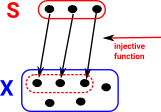It would not work to have a map in the other direction because elements in S are not uniquely identified with elements in X.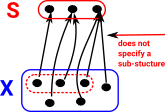However we can define a subset with a map going out of X. This maps onto a two point set 2 or {0,1}. Those elements that are in the subset map to 1 and those that are not map to 0. This is called the characteristic function.We can complete the diagram by adding an object representing the 'true' element. All elements in S map to it. As we can see, the diagram commutes.So, in the general case for sets, we have this diagram which commutes. The diagram is also a pullback.The diagram relates the set category to the logic of boolean algebra but without the law of the excluded middle, that is intuitional or constructive logic.### Alternative Viewpoint

 We have two sets, 'A' and 'B', with an arbitrary mapping 'F' between them. We can define a subset of set 'B' by using the predicate 'P'. For every subset of 'B' defined by 'P' there exists a subset of 'A' defined by 'R'.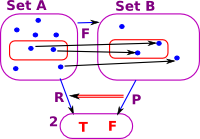However this does not necessarily work in the other direction. Here is an example of a subset of 'A' which does not map to a subset of 'B'.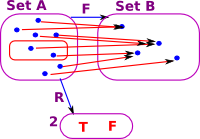So the map 'F' between objects induces a contravarient map between arrows P->R.

### Generalisation to Non-sets

We can genarise from elementary topos based on sets to other topoi.

 We generalise this to categories that are not sets. X becomes some category and S becomes a sub-category. The injective mapping becomes a monic arrow. The characteristic function then becomes the sub-object classifier.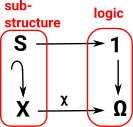### Graph Theory

Graph theory is an example of a category with a logic not based on sets. Possible values for Ω in a graph:

 For nodes: For edges: Is not in subgraph Is in subgraph Is in subgraph Not in subgraph but its source and target are. Not in subgraph but its source is. Not in subgraph but its target is. Not in subgraph and neither is its source or target.
 Graphical representatin of Ω: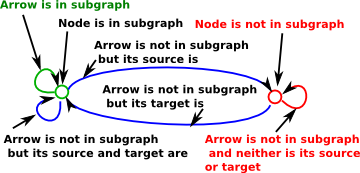### Subobject Classifier

See page here for a non-categorical approach to sub-objects.If the arrow 'f' is monic (injective) then it maps to a subobject (subset) of 'B'. That is: there is a subobject of B that is isomorphic to A.

The inclusion relation on subobjects is:

• reflexive
• transitive

In order to define the subobject we must define the monic 'upto equality' which is not in the spirit of category theory. We will go on to define a 'subobject classifier' which defines a subobject, in a much more category theoretic way, by the composition of maps (and also relates the whole subject to logic).

#### Naming Arrows

In category theory we don't usually identify elements, such as elements of a set, because we only tend to determine things 'upto isomorphism'. The objects in a category are whole 'structures', they don't represent individual elements.

However, there is the possibility to indicate elements indirectly using arrows. For instance, if we want to enter a specific element we can use:

1A

because, in sets, 1 is the single element set so it will indicate an element uniquely.

We can also use naming arrows. An arrow:

f: AB

Is a subset of a function spacef:{0}BAfis called the 'name' of the function.

#### Classifier

In the opposite direction there is a correspondence between subsets of B and functions:

B 2

This is in the space 2B which corresponds to the powerset(B), that is, all the possible subsets of B.

 The characteristic arrow: xf classifies objects of B to determine if they are images of A. This is a pullback square. The object 2 has two values: 0 and 1. We also use the symbol Ω and call the elements 'true' and 'false'.This means the topos theory is related to logic. (see also 'characteristic function' in number theory).

#### Example in Set

Example in set
Set A (blue) is subset of B (red).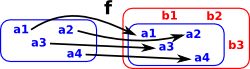If we take the inverse of f we get the concept of a 'bundle' as discussed on this page.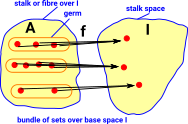classifier for set.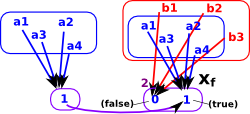## Predicate Category

The relationship SX above is a predicate. We can form a category of predicates on sets as follows:

 Objects predicates are pairs (S,X) where SX X(i) implies an element iS is a free variable in S. Morphisms (S,X) -> (T,Y) where: u:S-> T and X(i) implies: Y(u(i))### Family of Sets

A family of sets consists of an index set I and, for each element k of I, a set Sk.

There seems to be two ways to conceptulise this:

• As a 2-category. A set of sets.
• As disjoint sets.

### Modest Sets

Set theoretic model of polymorphism.

### Indexed Categories

(fibred category)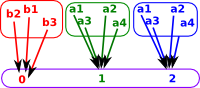example

many sorted algebra ∑

### Generalisation from Sets to Categories

Here we have illustrated some basic concepts using sets but they can be generalised to categories as shown here:

Sets Categories

Injective Function

No two distinct inputs give the same output.

We can define 'A' a subset of 'B' by using an injective function from CMonic Arrow

Whenever f•g = f•h then g=h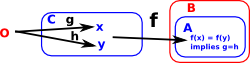Here we are showing this as an inclusion function because we are interested in subset classifiers.

Injective function f: C >->B determines A as a subset of B (AB)
Im f = {f(x): xC}
where Im = Image

Set inclusion is a partial odering on the Power set

Surjective function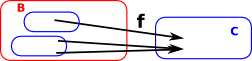Epic ArrowInverse Functions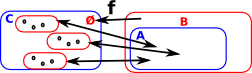Power SetPower Object

subobjects of d form a poset
(Sub(d),)

## Topological Space

In many cases the concept of a metric space is unnessary, however we still need the concept of 'nearness' and hence 'continuity'. 'Topological space' based on the 'topological open set' is the most general way we can do this. This allows us to define nearness purely using the concept of a subset.

#### Hausdorff Space

Hausdorff space is a bit more specific than general topological space. Space is Hausdorff if, in addition to being a topological open set, for any two points:
x1, x2X there are disjoint open sets U1, U2 that contain x1and x2.

#### Basis

A basis is a subcollection B= U

where

• U is the open set
• B is the basis
•= a subcollection (subset) - I need to add the proper symbol for this.

such that evey element of U is a union of open sets in B.

Topos

 These lectures are about physics using topos theory. It does not even get to topos until 5. parts 1-4 contains lots of other defintions.

Where I can, I have put links to Amazon for books that are relevant to the subject, click on the appropriate country flag to get more details of the book or to buy it from them.The Princeton Companion to Mathematics - This is a big book that attempts to give a wide overview of the whole of mathematics, inevitably there are many things missing, but it gives a good insight into the history, concepts, branches, theorems and wider perspective of mathematics. It is well written and, if you are interested in maths, this is the type of book where you can open a page at random and find something interesting to read. To some extent it can be used as a reference book, although it doesn't have tables of formula for trig functions and so on, but where it is most useful is when you want to read about various topics to find out which topics are interesting and relevant to you.